• ## Recent Commentsbob on Proof that Humans Are Evi…Mike on Viking Metal Meets Viking…sir on Set Algebra: A Quick Reference…Crystal on Proof that Humans Are Evi…Felicis on Viking Metal Meets Viking…
• Advertisements

## Set Algebra: A Quick Reference Guide

[The following set-algebra laws are “borrowed”, in the great tradition of the internet, from Wikipedia.  If you would like more background on why these things work, the article goes into more detail, and of course has the complete lattice of links to accompany it.]

I have a god-awful memory.  So, I’m constantly struggling to find some basic property of sets whenever I’m trying to prove something that I KNOW would be easy, if I could just remember the damned law.  Well, after scouring the net, Wikipedia’s version turned out to be best … but I don’t need all the exposition, just the laws spelled out, easy to see.

Like to here it, here it go:

PROPOSITION 1: For any sets A, B, and C, the following identities hold:

commutative laws:

•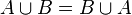$A cup B = B cup A,!$
•$A cap B = B cap A,!$
associative laws:

•$(A cup B) cup C = A cup (B cup C),!$
•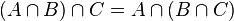$(A cap B) cap C = A cap (B cap C),!$
distributive laws:

•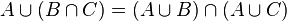$A cup (B cap C) = (A cup B) cap (A cup C),!$
•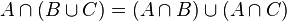$A cap (B cup C) = (A cap B) cup (A cap C),!$

PROPOSITION 2: For any subset A of universal set U, the following identities hold:

identity laws:
•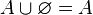$A cup varnothing = A,!$
•$A cap U = A,!$
complement laws:

•$A cup A^C = U,!$
•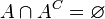$A cap A^C = varnothing,!$

PROPOSITION 3: For any subsets A and B of a universal set U, the following identities hold:

idempotent laws:

•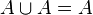$A cup A = A,!$
•$A cap A = A,!$
domination laws:

•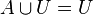$A cup U = U,!$
•$A cap varnothing = varnothing,!$
absorption laws:

•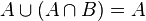$A cup (A cap B) = A,!$
•$A cap (A cup B) = A,!$

PROPOSITION 4: Let A and B be subsets of a universe U, then:

De Morgan’s laws:

•$(A cup B)^C = A^C cap B^C,!$
•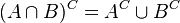$(A cap B)^C = A^C cup B^C,!$
double complement or Involution law:

•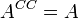$A^{CC} = A,!$
complement laws for the universal set and the empty set:

•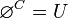$varnothing^C = U$
•$U^C = varnothing$

PROPOSITION 5: Let A and B be subsets of a universe U, then:

uniqueness of complements:

• If$A cup B = U,!$, and$A cap B = varnothing,!$, then$B = A^C,!$

PROPOSITION 6: If A, B and C are sets then the following hold:

reflexivity:

•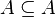$A subseteq A,!$
antisymmetry:

•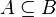$A subseteq B,!$ and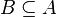$B subseteq A,!$ if and only if$A = B,!$
transitivity:

• If$A subseteq B,!$ and$B subseteq C,!$, then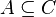$A subseteq C,!$

PROPOSITION 7: If A, B and C are subsets of a set S then the following hold:

existence of a least element and a greatest element:

•$varnothing subseteq A subseteq S,!$
existence of joins:

•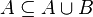$A subseteq A cup B,!$
• If$A subseteq C,!$ and$B subseteq C,!$, then$A cup B subseteq C,!$
existence of meets:

•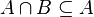$A cap B subseteq A,!$
• If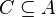$C subseteq A,!$ and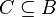$C subseteq B,!$, then$C subseteq A cap B,!$

PROPOSITION 8: For any two sets A and B, the following are equivalent:

•$A subseteq B,!$
•$A cap B = A,!$
•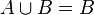$A cup B = B,!$
•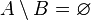$A setminus B = varnothing$
•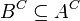$B^C subseteq A^C$

PROPOSITION 9: For any universe U and subsets A, B, and C of U, the following identities hold:

•$C setminus (A cap B) = (C setminus A) cup (C setminus B),!$
•$C setminus (A cup B) = (C setminus A) cap (C setminus B),!$
•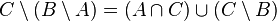$C setminus (B setminus A) = (A cap C)cup(C setminus B),!$
•$(B setminus A) cap C = (B cap C) setminus A = B cap (C setminus A),!$
•$(B setminus A) cup C = (B cup C) setminus (A setminus C),!$
•$A setminus A = varnothing,!$
•$varnothing setminus A = varnothing,!$
•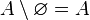$A setminus varnothing = A,!$
•$B setminus A = A^C cap B,!$
•$(B setminus A)^C = A cup B^C,!$
•$U setminus A = A^C,!$
•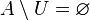$A setminus U = varnothing,!$
Advertisements

## Why Order is Important…

Not a lot of time this morning, but if you are interested in seeing how order is used in set theory, John Armstrong is doing a nice series of the creation of numbers at The Unapologetic Mathematician. Today he talks about Archimedean fields and how the real numbers are in the ‘sweet spot’ between being too big (any larger and they wouldn’t be Archimedean) and too small (any smaller and they would not be Dedekind Complete).

## Speaking of Set Theory

So, while I implied I would talk a little about Categories, since we just had our Set Theory final Exam (Saij and I), I thought perhaps a little discussion of this would be in order… Forgive the pun.

Indeed, while the basics of set theory were covered as always: looking at sets; their union and intersection; products of sets; and so forth, we also covered ordered sets. When order is dragged into the picture, we start to get a lot of interesting ideas that follow.